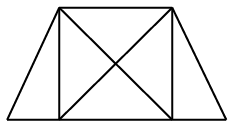Question 17

# How many triangles are there in the given figure ?Solution

Smaller triangles = 6

Triangles formed by combination of 3 triangles = 2

Larger triangles (at the diagonals) = 4

Thus, total triangles = 6 + 2 + 4 = 12

=> Ans - (C)

• Free SSC Study Material - 18000 Questions
• 230+ SSC previous papers with solutions PDF